# Realistic Raytracing

Zack Waters

## Introduction

For image synthesis we need to have some model of light.  The prevalent models of light are geometric optics and physical optics.  Geometric optics models light propagation in terms of rays that travel in straight lines and their paths are described through a series of reflections and refractions.  Physical optics treats light propagation as a wave phenomenon where effects of polarization, interference and diffraction can be modeled.

Classical raytracing, an application of geometric optics, point samples the incoming radiance from a scene by tracing rays from the eye through the scene to the lights.  The images generated by an implementation of the classical raytracing algorithm can look wonderful but also unrealistic and often times suffer from severe aliasing problems.  The problem is that the algorithm severely undersamples the domains for the integral equations that describe the complex optical interations of light.

Distribution raytracing, originally called distributed raytracing, extends classical raytracing by incorporating Monte Carlo techniques.  Instead of sampling with one ray it distributes multiple rays to sample the integrals over the pixel area, lens area, time, and the hemispheres for reflection and refraction.  This simple extension is much more computationally expensive but as a point sampling process it is necessary to render cool effects such as soft shadows, depth of field, motion blur, glossy and translucent surfaces.  Although distribution raytracing is a huge improvement it does not solve the complete problem.Cornell Box - Direct Lighting 16 samples were used per pixel, shadows and reflections.

For realistic image synthesis we also need to solve the rendering integrals for the light paths that account for global illumination and caustics.  However, these integrals can be deeply nested and seemingly impossible to solve.  Using Monte Carlo techniques we have the facilities for approximating these integrals.  The basic idea behind Monte Carlo techniques is to approximate a function by randomly sampling it within some domain.  Hopefully, the samples give some insight on what the functions look like.

Path tracing was developed as a solution to the complete rendering equation and is heavily based on Monte Carlo techniques.  It completely samples the entire domain while distribution raytracing only samples portions of it.  Path tracing renders a scene by tracing rays from the eye back to the light sources.  Each of these ray paths is a non-branching path where at each ray-surface interaction the new direction for the path is determined probabilistically.  For the solution to converge to the correct result many rays are needed.  The main problem with path tracing is that the variance in the solution shows up as noise.  Fortunately, there are ways to alleviate this noise such as using Photon Mapping to handle caustics.

In the following sections I will discuss the rendering equation, distribution raytracing, and will briefly touch upon path tracing.  This write-up reflects my understanding of these topics and there may be errors.  If there are any please let me know.

## The Rendering Equation

Introduced by James Kajiya in 1986, the rendering equation describes the transport of light from one surface point to another as the sum of emitted radiance and reflected radiance.where: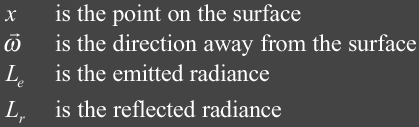Radiance tells us how much light energy is arriving or leaving a surface in a particular direction within some time frame.  In a vacuum radiance is constant along a line of sight which is an important property that makes raytracing possible.   The term that is the most interesting is the reflected radiance term, Lr, and can be described as the following:This integral takes into account all of the incoming light and computes the reflected light.  It takes into account all light paths such as those that contribute to caustics and global illumination.  The next several sections contains the details of this integral.

### Term### :  BRDF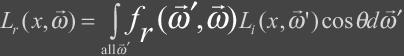The bidirectional reflectance distribution function (BRDF) describes how a surface reflects light energy.  Reflectance is the fraction of incident light that is reflected.  The simplified form of the BRDF is a 4D function of incident and outgoing directions.  The BRDF is given by the following ratio.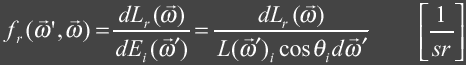where:For a BRDF to be physically plausible it must follow the law of energy conservation and must obey the Helmholtz reciprocity principle.  Following the law of energy conservation the BRDF should evaluate to a real number in the range [0, 1].  To be more specific the differential BRDF integrated over a hemisphere must be less then or equal to one.  This means we can not reflect more light then we have received.  This can be expressed mathematically as follows: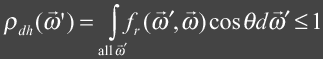This expression is also known as the directional hemispherical reflectance and describes the total amount of incident light energy that is reflected which must be less then or equal to 1.  Helmholtz reciprocity principle means that the sampling incident and reflected directions of the BRDF can be switched and the result will be the same.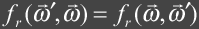Obtaining a BRDF for a material can be done in several ways.  One can obtain a BRDF through empirical measurements and then fit a mathematical function to the data.  Some examples of these empirical models are the popular Lambert, Phong, Blinn-Phong and Ward models.  The BRDF for a perfectly lambertian (diffuse) surface is just a constant as light is equally reflected in all directions.  Physically based models can be developed analytically and are rooted in physics.  Examples of these are Cook-Torrance model and a model presented by Kajiya in 1985 that handles anisotropic reflection.

### TermThe function Li describes the incident radiance at x.  The function may be a deeply nested integral equation as incident light may be indirectly reflected from other surfaces in the environment including itself.

### Term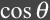### :  Cosine Law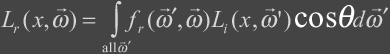For this term we are taking the dot product of the incident direction and normal to project the differential area subtended by the solid angle onto the base of the hemisphere as seen by x.  This term is necessary to account for Lambertĺs cosine law.  For a better understanding we have the following: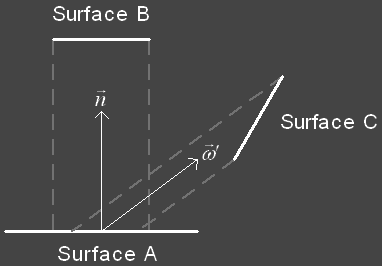Surface A is equally illuminated by surfaces B and C which both have the same surface area.  However, A can see more of B then it does of C because the projected area of C onto A is smaller then that of B.

### Term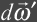### :  Differential Solid AngleThe differential solid angle is the differential quantity of choice when integrating over a hemisphere.  The solid angle generalizes a range of directions through a differential area on the hemisphere.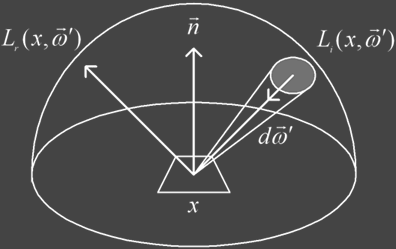## Solving the Rendering Equation

Two main approaches to solving the rendering equation are finite element methods and point sampling techniques.  For the rest of this paper we will only discuss point sampling techniques using Monte Carlo methods.

### Distribution Raytracing

Even before Kajiya formalized the rendering equation, Cook et al recognized that rendering is just the process of solving a set of nested integrals.  Some examples of these integrals are the integral over the pixel area, over the lens area, over time, and over the hemispheres for reflection and transmission.  These integrals do not have an analytical solution that can be computed in finite time, so instead we use Monte Carlo techniques to solve for them.

Basic idea behind Monte Carlo integration is to compute an average of random samples of the integrand f(x).  The basic Monte Carlo integration can be described as:where:There are several forms of Monte Carlo integration techniques with varying random sampling strategies such as importance sampling and stratified sampling.  Peter Shirley shows that stratified sampling is often times far superior to importance sampling. 

Stratified sampling is also easy to implement and no prior knowledge of the signal is needed.  Basically, the domain is divided up into equal strata and then the sub-domains are sampled by jittering samples about their centers.At surface locations distributed raytracing does not completely integrate over the entire domain, the hemisphere, but instead only over light sources and about reflection and transmission rays.  Because of this distributed raytracing does not take into account all light paths such as indirect illumination and caustic effects.  Never the less distributed raytracing is computationally expensive.

### Sampling Pixels

Pixels have a finite area and represent a single color.  In classical raytracing we are sampling the incoming radiance from the scene once per pixel.From the start classical raytracing is grossly undersampling the scene.  Distributed raytracing extends classical raytracing to use stochastic sampling, a Monte Carlo method, to approximate the integral over the area of the pixel.A common technique to approximate this nested integral is to use a jittered grid, a form of stratified sampling, to sub-sample the pixel.  The basic idea is to divide the pixel area into a grid of equal size cells or stata and the sample points are generated by jittering the center point of each cell.The pixel color is calculated by firing rays from the eye point through the each of the jittered sample locations and then these samples are averaged.

click on images to enlargeNo Antialiasing 4 samples per pixel 16 samples per pixel

In a basic raytracer implementation shadows are very sharp and typically this is because point light sources are used.  In order to create soft shadows we need an area light source.  The figure below shows the anatomy of a shadow for both a point light source and an area light source.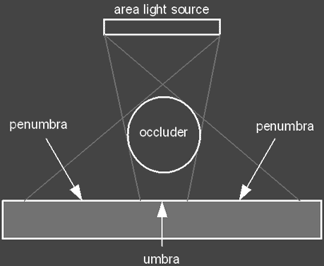The umbra is the region where the light is entirely occluded and the penumbra is the region where the light is partially occluded.

Soft shadows can be approximated in a standard raytracer by just placing several point light source near each other where each one has Nth the intensity of the base light they are approximating. Using this technique sharp transitions occur in the penumbra because we are still dealing with individual point light sources.  Here is an example where this technique is used.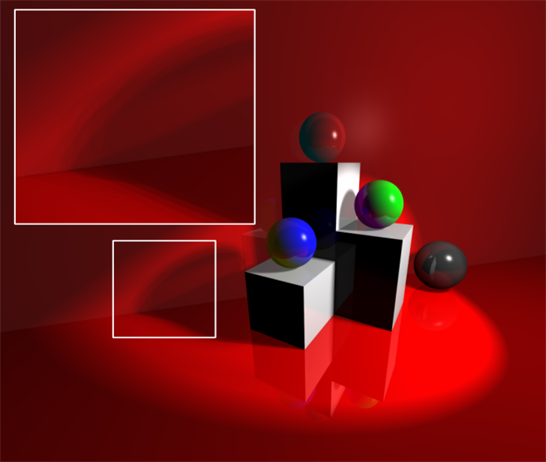RGBA Balls - Notice the sharp transitions in the penumbra.

Distributed raytracing resolves the problem by stochastically sampling area light sources.  To implement this we can add a shadow factor to our rendering equation that is a number between 0 and 1.  A shadow factor of 0 means we are completely occluded (umbra) or 1 if we are completely unoccluded.  A factor in between 0 and 1 means we are partially occluded (penumbra).

The shadow factor is calculated for each light source by generating a set of random sample points that are well distributed across the surface of the light source and then distributing a set of shadow feeler rays to these sample point locations.Each shadow feeler ray, Si, either hits the light source in which case it is assigned a value of 1 or it doesnĺt in which case it is assigned a value of 0.  To calculate the lightĺs contribution we just take the average of the shadow feelers and multiply it by the lightĺs intensity.Generating the sample points over the area of the light source is done by generating a jittered grid for n samples and then mapping the grid sample locations to a set of locations on the surface of the light source.  The mapping for a square area light is straight forward.

click on images to enlargen=1 sample for shadows n=16 samples for shadows n=16 samples for shadows Increased light size to increase the spread of the shadows.  Also, had to dim the light down.

For other complex light types the mapping is not as simple.  However, Peter Shirley describes in an article called ôNonuniform random point sets via warpingö for Graphics Gems III several warping transformations including that for spheres, hemispheres and triangles.  These transformations can be used to map the jittered grid sample points to most complex light surfaces.  This of course assume the light radiates energy equally across its surface.

### Gloss

Glossy surfaces are specular surfaces that are somewhat rough.  Distribution raytracing tackles the problem by distributing a set of reflection rays by randomly perturbing the ideal specular reflection ray.  The spread of the distribution determines the glossiness where a wider distribution spread models a rougher surface.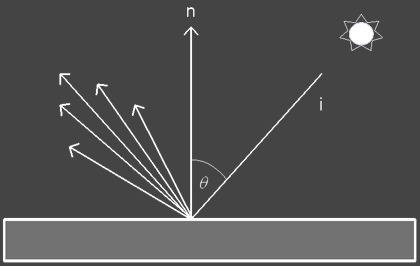To implement gloss you just need to setup a square that is perpendicular to the specular reflection vector and then map a jittered grid of sampling locations in which reflection rays are fired through.  The width of this square determines the glossiness of the surface.  In addition the ray contributions should be weighted using the BRDF or the same specular weighting function used on the ideal specular reflection rays.Alone this technique can only model very shallow bumpy surfaces such as a kitchen countertop where the distribution of the bumps is uniform.  However, we can incorporate a texture map that we look up into to determine the pattern of glossiness where each texel in the map is a gloss factor.  The gloss map can be used in addition to a bump map to model deeper groves.

 Glossy floor using 16 secondary specular rays

### Translucency

Transluceny is implemented in a similar manner as gloss.  Once the bulk of the implementation is complete for gloss it is straight forward to slightly modify it to include translucency.  The only difference is that instead of perturbing the ideal specular reflection ray you are now perturbing the ideal specular transmission ray in order to generate a set of secondary transmission rays.  A wider distribution of transmission rays will make the glass like material appear rougher.  Effects such as frosted gloss can be achieved.  For a wider range of effects this technique can be mixed with translucent and refraction maps.

### Depth of Field

The depth of field is the distance that objects appear in focus.  It is necessary to model a camera with a lens system to achieve depth of field effects.  For basic camera operation you need an opaque contain that contains a single aperture (opening).  This aperture controls the amount of light that may strike the image or film.  Light passing through the aperture is the only light allowed to strike the film.  The amount of time that the film is exposed to the light is controlled by a shutter.

In computer graphics the pinhole camera model is the most popular.  The film is enclosed in a box containing a pinhole aperture.  Everything in the scene is sharply focused onto the image plane.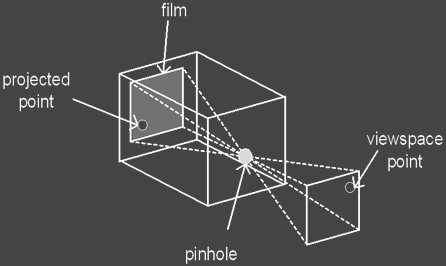The pinhole which is infinitely small becomes the focal point and the scene lies in front of it and is projected to the film which lies behind the pinhole.  In computer graphics the typical setup is that the image and scene lies in front of the focal point or point of projection.  Controlling the focal distance, the distance from the image plane to the point of projection, or the image dimensions effects the field of view but does not affect the focus of objects.  They will always appear in sharp focus.

A simple camera model for implementing depth of field is the thin lens camera model.  In this model the camera lens is a double convex lens of negligible thickness.  This means that light passing through the lens is refracted on a single plane called the principle plane instead of two refractions.  The lens has two focal points at equal distances in front and behind the lens.A phenomenon called the circle of confusion occurs when light from a single point is focused through a lens onto an image as a circle.  The reason that this occurs is because the focal distance needed to project the light as a single point is either in front or behind the image plane.  Remember light leaves a surface in all directions about the hemisphere and thus the circle is formed because different parts of the lens are focusing all light that it receives from the single surface point.  In the figure below light from the point on the focal plane is projected as a circle on the image plane with distance C.Given a focal length, f, and a distance, i, which is the distance from the pixel to the center of the lens, we can compute the depth of field effects by first computing the distance, s, from the center of the lens to a focal plane where all points will be in focus.  Using the thin-lens approximation we can find s.We now need to find a point P on the focal plane at distance s that is in the line of sight from the center of the lens to the pixel sample location.  Once point P is calculated we can generate a set of sample locations on the lens, again using a jittered grid, from which we trace rays from to point P.  We can then average the contribution from these rays to find the color at the pixel. However, since we are also sub-sampling the pixel we should pair samples from the pixels to samples on the lens.  To reduce alias artifacts we need to randomly pair the samples for each pixel to locations on the lens.  Peter Shirley discusses this in a tad more detail in his Realistic Raytracing book.Zero Uno Toys - http://www.splutterfish.com

### Motion Blur

Motion blur occurs because the shutter allows the film in the camera is exposed to light for a period of time.  While the shutter is opened any animation in the scene is captured on a single piece of film.  The result is an image where objects in motion are blurred.

To implement this multiple rays from the pixel are fired into the scene at different times and the contribution is averaged.  When taking into account depth of field, the computation can become more costly.  To reduce the computation the samples in time can be paired with pixel and lens samples.  Then the rays traced through these dimensions are then averaged for each pixel.

## Path Tracing

Path tracing was introduced by Kajiya in the same paper that he formalized the "The Rendering Equation".  He developed path tracing as a solution to the rendering the equation.  It handles indirect lighting as well as caustics.  In a path tracer you are tracing a single path from the eye back to the light source.  Instead of distributing mulitple secondary rays at each intersection, a single new ray path is chosen probabilistically.

Kajiya points out that first generation rays as well as light source rays are most important in terms of variance that they contribute to the pixel integral.  Secondary rays effect contribute less.  When you are only tracing a single path there needs to be a heuristic that tells the algorithm where the important sampling directions are.  Typically path tracers incorporate an importance function that basically describe what parts of the domain are important.  Sample paths are weighted according to their importance or contribution. We do not need to solve for the entire domain as the overall result will not look much different.  For example, we only need an accurate solution of the radiance equation for visible surfaces with significant contribution.  Importance needs to be propagated through the environment.  If a surface is important then any surfaces that contributes significantly to that surface is also important.

One major problem with path tracing is that variance shows up as noise in the final render.  The main contributor to noise is caustics and can be alleviated by using other techniques such as photon mapping.

click to enlarge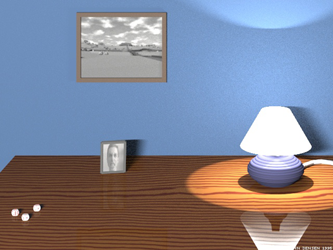1000 samplers were used per pixel. [Henrik Jensen] 2000 samples per pixel. Jensen states that render time was approximately 30 hours running on 30 SGI workstations. [Henrik Jensen]

## References

  Shirley, Peter Realistic Raytracing A K Peters, Ltd. Massachusetts, 2000.  Robert L. Cook, Thomas Porter, and Loren Carpenter.Distributed Raytracing.  Computer Graphics, 18(4):165-174, July 1984.  ACM Siggraph ĺ84 Conference Proceedings  James T. Kajiya.  The Rendering Equation.  Computer Graphics, 20(4):143-150, August 1986. ACM Siggraph ĺ86 Conference Proceedings.  T. Whitted. An improved illumination model for shaded display.  CACM, 23(6):343-349, June 1980.  Glassner, Andrew An Introduction to Ray Tracing California: Academic Press Limited, San Diego, 1989  Glassner, Andrew Principles of Digital Image Synthesis Morgan Kaufmann Publishers, Inc., San Fransico, 1995  Shirley, Peter Fundamentals of Computer Graphics A K Peters, Ltd, Massachusetts, 2002  Jensen, Henrik W. Realistic Image Synthesis Using Photon Mapping. A K Peters, Ltd, Massachusetts, 2001  W. Heidrich, Ph. Slusallek, and H.-P. Seidel. An Image-based Model for Realistic Lens Systems in Interactive Computer Graphics.  Giancoli, Douglas Physics for Scientist and Engineers. Prentice Hall, New Jersey, 1989